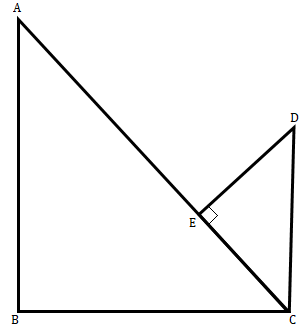"
">

# In figure below, if $AB\ ÔŐą\ BC$, $DC\ ÔŐą\ BC$, and $DE\ ÔŐą\ AC$, prove that $╬ö\ CED\ Ôł╝\ ╬ö\ ABC$."

Given:

In the given figure $AB\ ⊥\ BC$, $DC\ ⊥\ BC$, and $DE\ ⊥\ AC$.

To do:

We have to prove that $Δ\ CED\ ∼\ Δ\ ABC$.

Solution:

In $\vartriangle ABC$ and $\vartriangle CED$,

$\angle BAC+\angle BCA=90^o$

$\angle BCA+\angle ECD=90^o$

This implies,

$\angle BAC=\angle ECD$

In $\vartriangle ABC$ and $\vartriangle CED$,

$\angle ABC=\angle CED=90^o$

$\angle BAC=\angle ECD$

Therefore,

$Δ\ CED\ ∼\ Δ\ ABC$.

Hence proved.

Updated on: 10-Oct-2022

28 Views